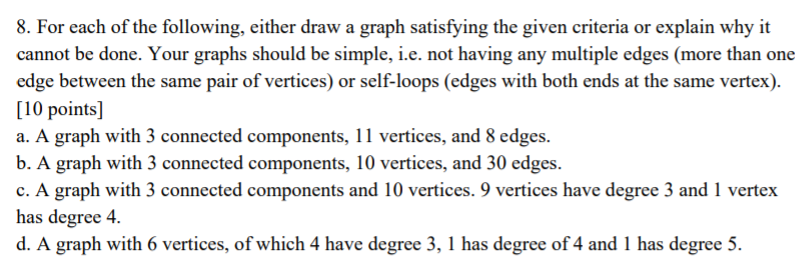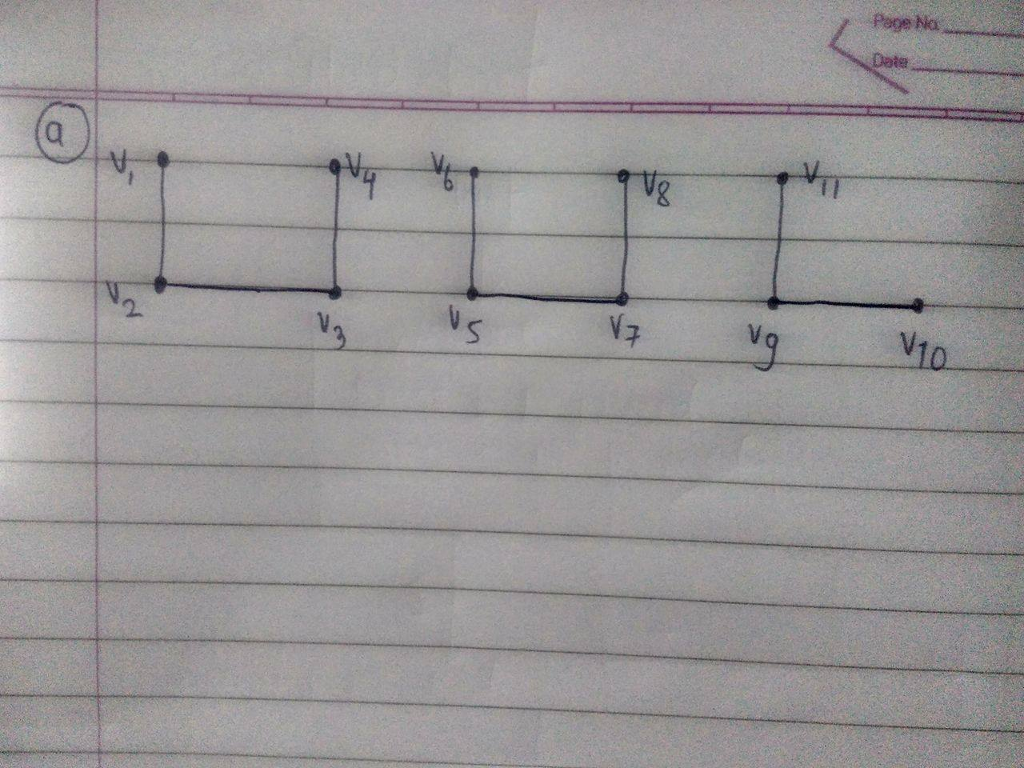# Question & Answer: For each of the following, either draw a graph satisfying the given criteria or explain why it cannot b…..NOTE: Please provoide the clear pictures and step by step if possible. Thanks

For each of the following, either draw a graph satisfying the given criteria or explain why it cannot be done. Your graphs should be simple, i.e. not having any multiple edges (more than one edge between the same pair of vertices) or self-loops (edges with both ends at the same vertex) a. A graph with 3 connected components, 11 vertices, and 8 edges. b. A graph with 3 connected components, 10 vertices, and 30 edges. c. A graph with 3 connected components and 10 vertices.9 vertices have degree 3 and 1 vertex has degree 4. d. A graph with 6 vertices, of which 4 have degree 3, I has degree of 4 and 1 has degree 5.

Don't use plagiarized sources. Get Your Custom Essay on
Question & Answer: For each of the following, either draw a graph satisfying the given criteria or explain why it cannot b…..
GET AN ESSAY WRITTEN FOR YOU FROM AS LOW AS \$13/PAGE

a) The graph with 3 connected components, 11 vertices, and 8 edgesb)

There does not exists any graph which satisfies given conditions. Because, with 10 vertices, we can have maximum 10C2 = 45 edges, but there will be a single connected component in the graph. For having 3 connected components we need pull atleast 2 vertices out which are not connected with each other and other 8 vertices. In that case we can have atmax 8C2 = 28 edges. And in other situations, number of edges are lesser than 28. So,There does not exists any graph which satisfies given conditions.

c)

We know the theorem “Sum of degrees of all vertices in the graph is even and is equal to twice the number of edges in the graph.”

But for the given situation, sum of degrees of all the vertices is equal to [9*(3) + 4 = 31]. So Given graph does not exists.

d)

We know the theorem “Sum of degrees of all vertices in the graph is even and is equal to twice the number of edges in the graph.”

But for the given situation, sum of degrees of all the vertices is equal to [4*(3) + 4 + 5 = 21]. So Given graph does not exists.